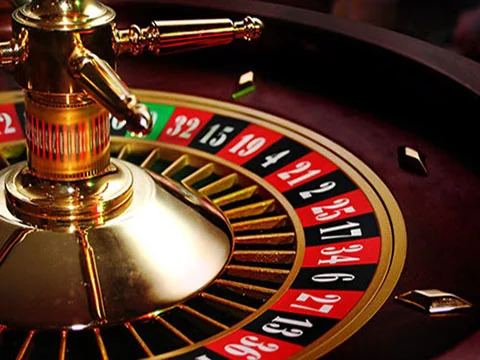## Mathematical method for calculating the expected value in rouletteAn interesting exhibition that explains the mathematical method with which the chances of winning the American roulette in the casino are calculated

The video proposed explains in a simple but technically correct way how to calculate the expected value ("Expected Value") of Roulette game In the American version, available both in traditional American casinos and in all online casinos, although not very practiced due to the unfavorable probability compared to the European counterpart.

This video serves to make the general concept understand, but remember that in any case, where available, it is always better to place bets in the European roulette: having only one zero, instead of a zero and a double zero, the probability in favor of the casino are minor null The same concepts can also be applied to roulette with a single zero, obviously the resulting value resulting will be slightly different.

The video is in English but easy to understand for those with a minimum knowledge of the language. For those who have problems with English, after the video a textual disquisition in American is available.

### How to calculate the expected value to roulette

A roulette has 38 numbers, 18 are red, 18 are black and two represent the zeros (green).

For the record, I am describing an American roulette. European roulette has only 1 zero.

The simplest appearance of roulette is to be able to focus on a color, let's say red. Point 1 dollar on red. It is an episode with equal probability, which means that if you bet 1 dollar, you can win 1 dollar or lose a dollar, based on the color of the number that comes out.

Let's try. Red! 27, I won! Now, what are the chances of winning? We have 38 numbers, each of which has the same probability of sorting and 18 of these are red. So the probability of winning is 18/38 = 0.473 = 47.3%.

Clearly you are disadvantaged in this game (compared to the counter, editor's note). We can quantify this disadvantage using the important concept of "expected value", that is, the weighted average of what you can win or lose.

When you bet a dollar on red, you have a probability of winning equal to 18/38 or a probability of losing 20/38, or of winning a "negative" dollar. The expected value is 1*(18/38) + (-1)*(20/38) = -0.526. This means that on average you lose 5.26 cents (0.0526 dollars) for each dollar bet

In the roulette you can also bet on other things in addition to color. For example, you can bet on a number between 1 and 12 (first dozen, ed.). We focus a dollar on the first dozen. The casino pays 2 to 1 this bet, which means that pointing 1 dollar in case of winning the casino pays \$ 2. Let's calculate the expected value: the probability of winning \$ 2 is 12/38, that of losing 1 dollar of 26/38. The expected value for this bet is 2*(12/38) + (-1)*(26/38) = -0.526, the same value as the previous episode!

Now, suppose to focus on a single number. The casino pays 35 to 1. pointing a dollar you are 1/38 probability of winning 35 and 37/38 to lose 1. When calculating the expected value of 35*(1/38) + (-1)*(37/38) , once again the result is -0.526. It is interesting to note that when you play roulette practically every bet has exactly the same expected value of about 5.3 cents in negative for each dollar bet.